# NCERT Exemplar solutions for Class 11 Physics chapter 5 - Laws of Motion [Latest edition]

#### Chapters## Solutions for Chapter 5: Laws of Motion

Below listed, you can find solutions for Chapter 5 of CBSE NCERT Exemplar for Class 11 Physics.

Exercises
Exercises [Pages 29 - 37]

### NCERT Exemplar solutions for Class 11 Physics Chapter 5 Laws of Motion Exercises [Pages 29 - 37]

#### MCQ I

Exercises | Q 5.1 | Page 29

A ball is travelling with uniform translatory motion. This means that ______.

• it is at rest.

• the path can be a straight line or circular and the ball travels with uniform speed.

• all parts of the ball have the same velocity (magnitude and direction) and the velocity is constant.

• the centre of the ball moves with constant velocity and the ball spins about its centre uniformly.

Exercises | Q 5.2 | Page 29

A metre scale is moving with uniform velocity. This implies ______.

• the force acting on the scale is zero, but a torque about the centre of mass can act on the scale.

• the force acting on the scale is zero and the torque acting about centre of mass of the scale is also zero.

• the total force acting on it need not be zero but the torque on it is zero.

• neither the force nor the torque need to be zero.

Exercises | Q 5.3 | Page 30

A cricket ball of mass 150 g has an initial velocity u = (3hati + 4hatj) m s−1 and a final velocity v = - (3hati + 4hatj) m s−1 after being hit. The change in momentum (final momentum-initial momentum) is (in kg m s1)

• zero

• -(0.45 hati + 0.6 hatj)

• -(0.9 hati + 1.2 hatj)

• -5 (hati + hatj)

Exercises | Q 5.4 | Page 30

In the previous problem (5.3), the magnitude of the momentum transferred during the hit is ______.

• Zero

• 0.75 kg ms–1

• 1.5 kg ms–1

• 14 kg ms–1

Exercises | Q 5.5 | Page 30

Conservation of momentum in a collision between particles can be understood from ______.

• Conservation of energy.

• Newton’s first law only.

• Newton’s second law only.

• Both Newton’s second and third law.

Exercises | Q 5.6 | Page 30

A hockey player is moving northward and suddenly turns westward with the same speed to avoid an opponent. The force that acts on the player is ______.

• frictional force along westward.

• muscle force along southward.

• frictional force along south-west.

• muscle force along south-west.

Exercises | Q 5.7 | Page 30

A body of mass 2 kg travels according to the law x(t) = pt + qt2 + rt3 where p = 3 ms−1, q = 4 ms−2 and r = 5 ms−3. The force acting on the body at t = 2 seconds is ______.

• 136 N

• 134 N

• 158 N

• 68 N

Exercises | Q 5.8 | Page 31

A body with mass 5 kg is acted upon by a force F = ( –3hati + 4hatj) N. If its initial velocity at t = 0 is v = (6hati - 12hatj) ms–1, the time at which it will just have a velocity along the y-axis is ______.

• never

• 10 s

• 2 s

• 15 s

Exercises | Q 5.9 | Page 31

A car of mass m starts from rest and acquires a velocity along east v = vhati (v > 0) in two seconds. Assuming the car moves with uniform acceleration, the force exerted on the car is ______.

• (mv)/2 eastward and is exerted by the car engine.

• (mv)/2 eastward and is due to the friction on the tyres exerted by the road.

• more than (mv)/2 eastward exerted due to the engine and overcomes the friction of the road.

• (mv)/2 exerted by the engine.

#### MCQ II

Exercises | Q 5.10 | Page 31

The motion of a particle of mass m is given by x = 0 for t < 0 s, x(t) = A sin 4 pt for 0 < t < (1/4) s (A > o), and x = 0 for t > (1/4) s. Which of the following statements is true?

1. The force at t = (1/8) s on the particle is – 16π2 Am.
2. The particle is acted upon by on impulse of magnitude 4π2 A m at t = 0 s and t = (1/4) s.
3. The particle is not acted upon by any force.
4. The particle is not acted upon by a constant force.
5. There is no impulse acting on the particle.
Exercises | Q 5.11 | Page 31

In Figure, the co-efficient of friction between the floor and the body B is 0.1. The co-efficient of friction between the bodies B and A is 0.2. A force F is applied as shown on B. The mass of A is m /2 and of B is m. Which of the following statements are true?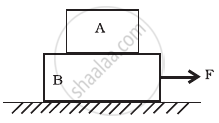1. The bodies will move together if F = 0.25 mg.
2. The body A will slip with respect to B if F = 0.5 mg.
3. The bodies will move together if F = 0.5 mg.
4. The bodies will be at rest if F = 0.1 mg.
5. The maximum value of F for which the two bodies will move together is 0.45 mg.
Exercises | Q 5.12 | Page 32

Mass m1 moves on a slope making an angle θ with the horizontal and is attached to mass m2 by a string passing over a frictionless pulley as shown in figure. The coefficient of friction between m1 and the sloping surface is µ.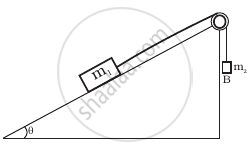1. If m2 > m1 sin θ, the body will move up the plane.
2. If m2 > m1 (sin θ + µ cos θ), the body will move up the plane.
3. If m2 < m1 (sin θ + µ cos θ), the body will move up the plane.
4. If m2 < m1 (sin θ − µ cos θ), the body will move down the plane.
Exercises | Q 5.13 | Page 32

In figure, a body A of mass m slides on plane inclined at angle θ1 to the horizontal and µ1 is the coefficent of friction between A and the plane. A is connected by a light string passing over a frictionless pulley to another body B, also of mass m, sliding on a frictionless plane inclined at angle θ2 to the horizontal. Which of the following statements are true?

1. A will never move up the plane.
2. A will just start moving up the plane when µ = (sin  θ_2 - sin  θ_1)/(cos  θ_1)
3. For A to move up the plane, θ2 must always be greater than θ1.
4. B will always slide down with constant speed.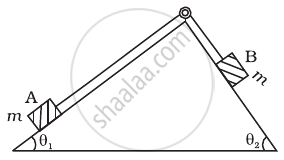Exercises | Q 5.14 | Page 32

Two billiard balls A and B, each of mass 50 g and moving in opposite directions with speed of 5 ms–1 each, collide and rebound with the same speed. If the collision lasts for 10–3 s, which of the following statements are true?

1. The impulse imparted to each ball is 0.25 kg ms–1 and the force on each ball is 250 N.
2. The impulse imparted to each ball is 0.25 kg ms–1 and the force exerted on each ball is 25 × 10–5 N.
3. The impulse imparted to each ball is 0.5 Ns.
4. The impulse and the force on each ball are equal in magnitude and opposite in direction.
Exercises | Q 5.15 | Page 33

A body of mass 10 kg is acted upon by two perpendicular forces, 6 N and 8 N. The resultant acceleration of the body is ______.

1. 1 m s–2 at an angle of tan−1 (4/3) w.r.t 6 N force.
2. 0.2 m s–2 at an angle of tan−1 (4/3) w.r.t 6 N force.
3. 1 m s–2 at an angle of tan−1 (3/4) w.r.t 8 N force.
4. 0.2 m s–2 at an angle of tan−1 (3/4) w.r.t 8 N force.

#### VSA

Exercises | Q 5.16 | Page 33

A girl riding a bicycle along a straight road with a speed of 5 ms–1 throws a stone of mass 0.5 kg which has a speed of 15 ms–1 with respect to the ground along her direction of motion. The mass of the girl and bicycle is 50 kg. Does the speed of the bicycle change after the stone is thrown? What is the change in speed, if so?

Exercises | Q 5.17 | Page 33

A person of mass 50 kg stands on a weighing scale on a lift. If the lift is descending with a downward acceleration of 9 ms–2, what would be the reading of the weighing scale? (g = 10 ms–2)

Exercises | Q 5.18 | Page 33

The position time graph of a body of mass 2 kg is as given in figure. What is the impulse on the body at t = 0 s and t = 4 s.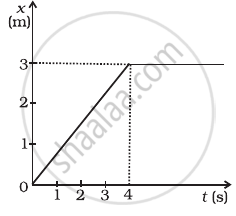Exercises | Q 5.19 | Page 34

A person driving a car suddenly applies the brakes on seeing a child on the road ahead. If he is not wearing seat belt, he falls forward and hits his head against the steering wheel. Why?

Exercises | Q 5.20 | Page 34

The velocity of a body of mass 2 kg as a function of t is given by v(t) = 2t  hati + t^2hatj. Find the momentum and the force acting on it, at time t = 2s.

Exercises | Q 5.21 | Page 34

A block placed on a rough horizontal surface is pulled by a horizontal force F. Let f be the force applied by the rough surface on the block. Plot a graph of f versus F.

Exercises | Q 5.22 | Page 34

Why are porcelain objects wrapped in paper or straw before packing for transportation?

Exercises | Q 5.23 | Page 34

Why does a child feel more pain when she falls down on a hard cement floor, than when she falls on the soft muddy ground in the garden?

Exercises | Q 5.24 | Page 34

A woman throws an object of mass 500 g with a speed of 25 ms1.

1. What is the impulse imparted to the object?
2. If the object hits a wall and rebounds with half the original speed, what is the change in momentum of the object?
Exercises | Q 5.25 | Page 34

Why are mountain roads generally made winding upwards rather than going straight up?

#### SA

Exercises | Q 5.26 | Page 34

A mass of 2 kg is suspended with thread AB (Figure). Thread CD of the same type is attached to the other end of 2 kg mass. Lower thread is pulled gradually, harder and harder in the downward directon so as to apply force on AB. Which of the threads will break and why?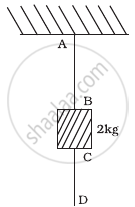Exercises | Q 5.27 | Page 34

In the above given problem if the lower thread is pulled with a jerk, what happens?

Exercises | Q 5.28 | Page 34

Two masses of 5 kg and 3 kg are suspended with help of massless inextensible strings as shown in figure. Calculate T1 and T2 when whole system is going upwards with acceleration = 2 ms2 (use g = 9.8 ms–2).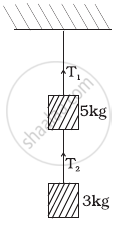Exercises | Q 5.29 | Page 35

Block A of weight 100 N rests on a frictionless inclined plane of slope angle 30° (figure). A flexible cord attached to A passes over a frictonless pulley and is connected to block B of weight W. Find the weight W for which the system is in equilibrium.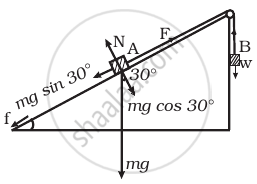Exercises | Q 5.30 | Page 35

A block of mass M is held against a rough vertical wall by pressing it with a finger. If the coefficient of friction between the block and the wall is µ and the acceleration due to gravity is g, calculate the minimum force required to be applied by the finger to hold the block against the wall?

Exercises | Q 5.31 | Page 35

A 100 kg gun fires a ball of 1 kg horizontally from a cliff of height 500 m. It falls on the ground at a distance of 400 m from the bottom of the cliff. Find the recoil velocity of the gun. (acceleration due to gravity = 10 ms–2)

Exercises | Q 5.32 | Page 35

Figure shows (x, t), (y, t ) diagram of a particle moving in 2-dimensions.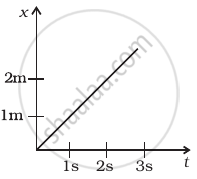(a)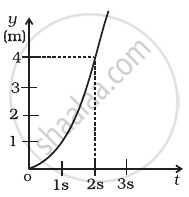(b)

If the particle has a mass of 500 g, find the force (direction and magnitude) acting on the particle.

Exercises | Q 5.33 | Page 35

A person in an elevator accelerating upwards with an acceleration of 2 ms–2, tosses a coin vertically upwards with a speed of 20 ms1. After how much time will the coin fall back into his hand? ( g = 10 ms–2)

#### LA

Exercises | Q 5.34 | Page 35

There are three forces F1, F2 and F3 acting on a body, all acting on a point P on the body. The body is found to move with uniform speed.

1. Show that the forces are coplanar.
2. Show that the torque acting on the body about any point due to these three forces is zero.
Exercises | Q 5.35 | Page 36

When a body slides down from rest along a smooth inclined plane making an angle of 45° with the horizontal, it takes time T. When the same body slides down from rest along a rough inclined plane making the same angle and through the same distance, it is seen to take time pT, where p is some number greater than 1. Calculate the co-efficient of friction between the body and the rough plane.

Exercises | Q 5.36 | Page 36

Figure shows (vx, t) and (vy, t) diagrams for a body of unit mass. Find the force as a function of time.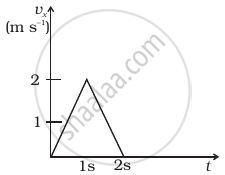(a)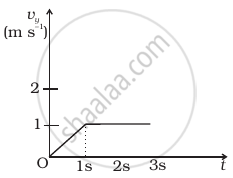(b)
Exercises | Q 5.37 | Page 36

A racing car travels on a track (without banking) ABCDEFA (Figure). ABC is a circular arc of radius 2 R. CD and FA are straight paths of length R and DEF is a circular arc of radius R = 100 m. The co-efficient of friction on the road is µ = 0.1. The maximum speed of the car is 50 ms–1. Find the minimum time for completing one round.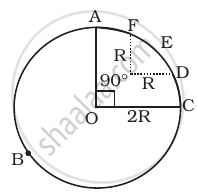Exercises | Q 5.38 (a) | Page 36

The displacement vector of a particle of mass m is given by r(t) = hati A cos ωt + hatj B sin ωt. Show that the trajectory is an ellipse.

Exercises | Q 5.38 (b) | Page 36

The displacement vector of a particle of mass m is given by r(t) = hati A cos ωt + hatj B sin ωt. Show that F = − mω2r.

Exercises | Q 5.39 | Page 37

A cricket bowler releases the ball in two different ways

1. giving it only horizontal velocity, and
2. giving it horizontal velocity and a small downward velocity. The speed vs at the time of release is the same. Both are released at a height H from the ground. Which one will have greater speed when the ball hits the ground? Neglect air resistance.
Exercises | Q 5.40 | Page 37

There are four forces acting at a point P produced by strings as shown in the figure, which is at rest. Find the forces F1 and F2.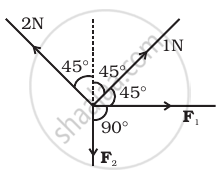Exercises | Q 5.41 | Page 37

A rectangular box lies on a rough inclined surface. The co-efficient of friction between the surface and the box is µ. Let the mass of the box be m.

1. At what angle of inclination θ of the plane to the horizontal will the box just start to slide down the plane?
2. What is the force acting on the box down the plane, if the angle of inclination of the plane is increased to α > θ ?
3. What is the force needed to be applied upwards along the plane to make the box either remain stationary or just move up with uniform speed?
4. What is the force needed to be applied upwards along the plane to make the box move up the plane with acceleration a?
Exercises | Q 5.42 | Page 37

A helicopter of mass 2000 kg rises with a vertical acceleration of 15 ms–2. The total mass of the crew and passengers is 500 kg. Give the magnitude and direction of the (g = 10 ms–2)

1. force on the floor of the helicopter by the crew and passengers.
2. action of the rotor of the helicopter on the surrounding air.
3. force on the helicopter due to the surrounding air.

## Solutions for Chapter 5: Laws of Motion

Exercises## NCERT Exemplar solutions for Class 11 Physics chapter 5 - Laws of Motion

Shaalaa.com has the CBSE Mathematics Class 11 Physics CBSE solutions in a manner that help students grasp basic concepts better and faster. The detailed, step-by-step solutions will help you understand the concepts better and clarify any confusion. NCERT Exemplar solutions for Mathematics Class 11 Physics CBSE 5 (Laws of Motion) include all questions with answers and detailed explanations. This will clear students' doubts about questions and improve their application skills while preparing for board exams.

Further, we at Shaalaa.com provide such solutions so students can prepare for written exams. NCERT Exemplar textbook solutions can be a core help for self-study and provide excellent self-help guidance for students.

Concepts covered in Class 11 Physics chapter 5 Laws of Motion are Lubrication - (Laws of Motion), Law of Conservation of Linear Momentum and Its Applications, Aristotle’s Fallacy, The Law of Inertia, Newton’s Second Law of Motion, Newton's Third Law of Motion, Equilibrium of a Particle, Common Forces in Mechanics, Circular Motion and Its Characteristics, Solving Problems in Mechanics, Static and Kinetic Friction, Laws of Friction, Inertia, Intuitive Concept of Force, Dynamics of Uniform Circular Motion - Centripetal Force, Examples of Circular Motion (Vehicle on a Level Circular Road, Vehicle on a Banked Road), Newton's First Law of Motion, Rolling Friction, Introduction of Motion in One Dimension, Conservation of Momentum.

Using NCERT Exemplar Class 11 Physics solutions Laws of Motion exercise by students is an easy way to prepare for the exams, as they involve solutions arranged chapter-wise and also page-wise. The questions involved in NCERT Exemplar Solutions are essential questions that can be asked in the final exam. Maximum CBSE Class 11 Physics students prefer NCERT Exemplar Textbook Solutions to score more in exams.

Get the free view of Chapter 5, Laws of Motion Class 11 Physics additional questions for Mathematics Class 11 Physics CBSE, and you can use Shaalaa.com to keep it handy for your exam preparation.